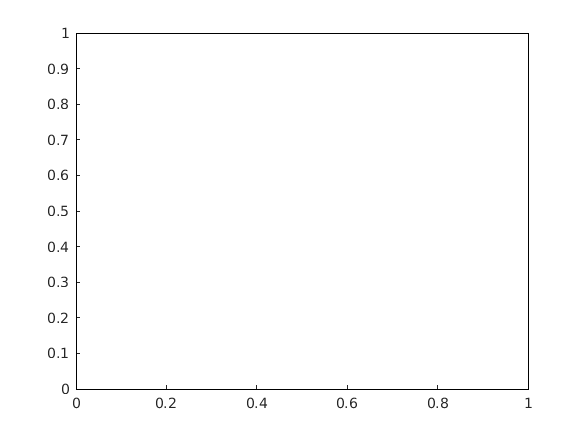Cody

# Problem 45583. Determined if input object array is heterogeneous

Solution 2338003

Submitted on 22 May 2020 by Zhuohe Liu
This solution is locked. To view this solution, you need to provide a solution of the same size or smaller.

### Test Suite

Test Status Code Input and Output
1   Pass
x = [figure; axes; rectangle]; y_correct = true; assert(isequal(isheterogeneous(x),y_correct))

``
2   Pass
x = [matlab.net.http.io.FileProvider, matlab.net.http.io.JSONProvider]; y_correct = true; assert(isequal(isheterogeneous(x),y_correct))

3   Pass
x = ""; y_correct = false; assert(isequal(isheterogeneous(x),y_correct))

4   Pass
x = rand(5, 4); y_correct = false; assert(isequal(isheterogeneous(x),y_correct))

5   Pass
x = {table(), 123, digraph()}; y_correct = false; assert(isequal(isheterogeneous(x),y_correct))

6   Pass
x = [figure; figure]; y_correct = false; assert(isequal(isheterogeneous(x),y_correct))

``
``
7   Pass
x = []; y_correct = false; assert(isequal(isheterogeneous(x),y_correct))